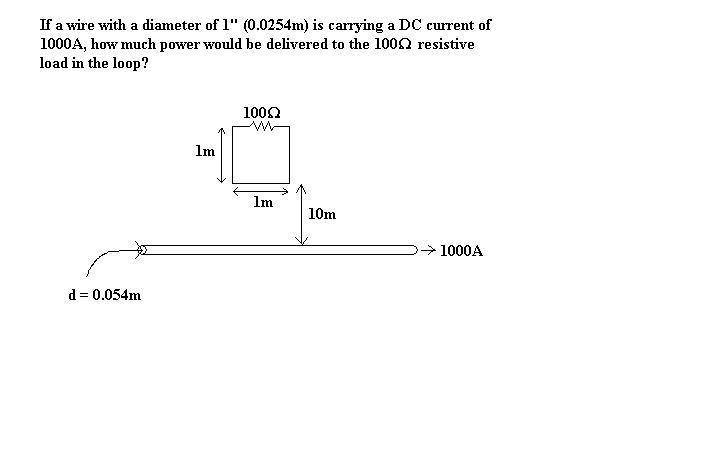# Power Delivered through a resistive load

Hey guys! I'm brand new to the forum and getting back into electromagnetics again. I had a question on one of my homework problems

## Homework Statement

If a wire with a diameter of 0.0254m is carrying a DC current of 1000A, how much power is delivered to the 100ohm resistive load in the loop.

(The picture shows an infinitely long wire carrying the current, and a square current loop with 1m sides floating above it 10m with a 100ohm resistor on one the top side of the loop)

## Homework Equations

I believe you are supposed to use the equation for Flux intensity and induced emf, but I'm not sure. I managed to calculate flux, but I'm not sure how to apply it in the given problem statement.

## The Attempt at a Solution

This is as far as I got, see attached image. I can't make the correlation. I think it has something to do with mutual inductance, but every formula/book I read has some time varying value.edit: Here's what I've done so far (whether or not it's right...no clue)
http://img10.imageshack.us/img10/5328/prob31.th.jpg [Broken]

Last edited by a moderator:

rude man
Homework Helper
Gold Member

## Homework Statement

If a wire with a diameter of 0.0254m is carrying a DC current of 1000A, how much power is delivered to the 100ohm resistive load in the loop.

## Homework Equations

I believe you are supposed to use the equation for Flux intensity and induced emf, but I'm not sure. I managed to calculate flux, but I'm not sure how to apply it in the given problem statement.

Has nothing to do with magnetic flux since it's dc current. The flux is there all right but does not affect dc current levels.

Calculate the resitance of the wire and go from there.

How are you supposed to calculate the resistance of the wire if the problem statement doesn't give you rho? It just gives me the current of the wire and its diameter of 1" that is 10m away from the current square loop containing the 100ohm resistor.

If flux isn't a part of it, I don't see how any power can be given to the floating wire loop above the current carrying conductor. It doesn't make sense to me :(

rude man
Homework Helper
Gold Member
How are you supposed to calculate the resistance of the wire if the problem statement doesn't give you rho? It just gives me the current of the wire and its diameter of 1" that is 10m away from the current square loop containing the 100ohm resistor.

If flux isn't a part of it, I don't see how any power can be given to the floating wire loop above the current carrying conductor. It doesn't make sense to me :(

You didn't say anything about the loop moving. If it's moving in some way, rotating or whatever, that presents an entirely different situation.

Please give us the exact and complete problem. A picture WOULD help. Can you maybe take a close-up photo of i,and send us the jpeg? Or draw it by hand, scan it & jpeg it to us?

I admit I should have smelled a rat when you didn't include a voltage source in your (question).

Sorry about before, I edited the original post with a picture. I tried to draw it in paint the best I could.

rude man
Homework Helper
Gold Member
If the picture is the complete problem, then the answer is zero.

The loop has to move somehow in order to get any power into the resistor.

Looks like you have a good grasp on how to get the magnetic flux. Unfortunately, emf = -d(flux)/dt and you have no d(flux)dt if it just sits there and looks pretty.

Thanks a ton. It was bothering me for a while that the professor would assign a trick question, go figure.

rude man
Homework Helper
Gold Member
He's probably a sadist. Most of us physicists/engineers are. :-)